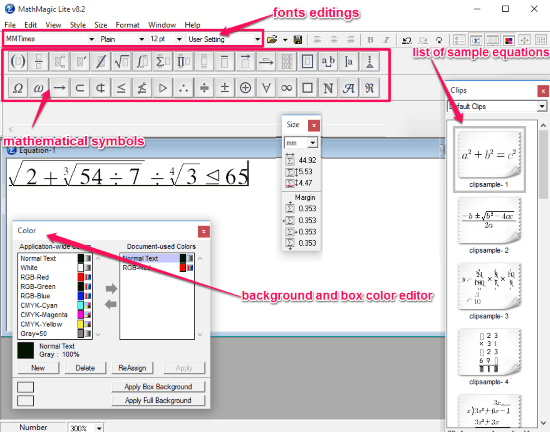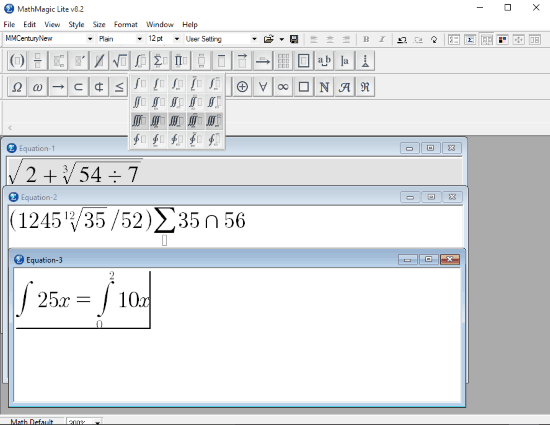Editor Ratings:
User Ratings:
[Total: 6 Average: 1.7]

MathMagic Lite is a versatile free math equation editor For Windows. It basically helps users to edit mathematical equation from the list of sample equations according to your need. You can also create a new equation by using all the mathematical symbols present in the toolbar. You can also provide separate Fonts, Font Style, and Font Size for Functions, Variables and Numbers. Once the equation is ready, you can copy it to clipboard and paste on any other application like WordPad, Microsoft Word, etc. These equations can be saved to your PC as MathMagic’s internal format, which can be later edited if needed. You can also save these equations as bitmap formats like JPG, PNG, GIF, etc.

Mathematics is like one of the main pillars of education, and math can’t sustain without equations. So for documenting mathematical equations on your PC, you will need a math equation editor as you can’t access all mathematical symbols from your keyboard. And, MathMagic Lite does a great job of math equation editor and that too for free.You can also take a look at these scientific calculators used for calculating complex equations:

## How To Use This Math Equation Editor Software:

MathMagic Lite is a free maths equation editor for Windows. However, its use is free only for personal use, and you will have to pay for its professional use. MathMagic Lite also works On Mac OS X, iOS and Android.

Once you have downloaded and installed the software from the link provided at the end of this review, you can start editing or creating math equations. All the useful tools needed for editing equations are available on its toolbar. And equations can be edited in the equation Window. You can use as many equation Windows as you need.For editing equations you can either use sample equations or your can create a new equation using all the different mathematical symbols available on the toolbar. For using symbols you will need to click on the relevant symbol icon and then select the symbol from the drop down. This way you will be able to access all the symbols in the toolbar.

Further you can use the different Fonts, Font Styles, and Font Sizes to represent different Functions, Variables, and Numbers in equations.

If your equations are multi line, then you can use the alignment tools to align them on left, center, or right. You also get to Undo and Redo your action.Further, you can use Equation Preferences tool to define spacing, style, size, and shape of Symbols. As I have mentioned earlier, using Color Window you can edit background color and box color (equation area) of the equation Window.

Once your equations are ready, you can simply select the equations and copy them to clipboard, so that you can paste it to any other software. Or you can also save them to your computer for future usage as bitmap formats or in MathMagic’s internal format.

## Verdict:

MathMagic Lite is a very useful freeware to edit mathematical equations and it works fine on its promises. So I would totally recommend this software to all those people in need of a free math equation editor.

 Editor Ratings: User Ratings:[Total: 6 Average: 1.7] Home Page URL: Click Here Works With: Windows, Mac OS X, Android, iOS Free/Paid: Free Tags: equationmathematics